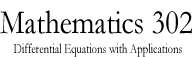Math 302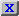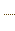HW3.pdfStatement on DisabilitiesStatement on EthicsHomeworkJava ToolsAn example of a circuitAn example of a circuitAn example of a circuitAn Application to Population DynamicsAn application to Population DynamicsSlope Field CalculatorODE 2D CalculatorODE 2D CalculatorODE 3D CalculatorODE 3D CalculatorSlope Field CalculatorSlope Field CalculatorSolution VerifierSolution Verifier 2DSolution Verifier 2DSolution VerifierA Lotke-Volterra SystemA Lotke-Volterra systemLabor Managed Oligopoly - Two firmsODE 3D Calculator2D Map CalculatorA model of sunamiA model of sunamiThe three body problemThe two body problemThe two body problemVan der Pol EquationVan der Pol EquationList of parameters of the JOde AppletBrowser test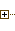Generated Documentation (Untitled)ODE 2D CalculatorUsing Marek Rychliks's Applet for Euler's MethodJOde Manual PageJOde - An Applet for Studying Ordinary Differential Equations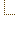ODE 2D CalculatorSyllabus
 Math Dept. Home Help room# Instructions for using the Differential Equations Applet

Go to the applet

## Entering differential equations

• Enter the right hand side of the equation into the area after
`eqn #k: dy/dx= `
The number k is used to identify the equation number in error messages, so that possible problems can be traced to a particular equation. In the default mode, the applet has only one equation. Equation #0, if present, contains initialization code. This code is evaluated only once after its change. It is typically used to define constants and functions.
• Any expression in variables x and y is allowed, using operators +,-,* and /, and mathematical functions from the following list: sin, cos, tan, arctan, arcsin, exp, ln, sqrt, abs, min, max (these last two are binary). Mathematical constants "E", "PI" and "Infinity" are also available. Examples of valid expressions:
• sin(x)/y
• y^2*sin(x)
• min(x,y)
• abs(x^2-y)
• sin(PI*y)
• exp(y)
• E^y
The applet supports user-defined functions, conditionals and loops described in this section.
• The equation is entered into the Applet by hitting Return or by pressing the "Submit All" button. Immediately after submission, the slope field and solutions are going to be plotted.

## Function reference

The following functions are available for use in expressions:
sin(x)
Returns sine of x.
cos(x)
Returns cosine of x.
tan(x)
Returns tangent of x.
artcan(x)
Returns inverse tangent of x.
arcsin(x)
Returns inverse sine of x.
abs(x)
Returns absolute value of x, i.e. x for x>=0 and -x for x< 0.
exp(x)
Returns the exponential of x.
ln(x)
Returns the natural logarithm of x.
sqrt(x)
Returns the square root of x for x>=0. If x< 0
min(x, y)
Returns x if x< y and y otherwise.
max(x, y)
Returns y if x< y and x otherwise.
step(x)
Returns 0 for x < 0 and 1 for x >= 0.
sgn(x)
Returns -1 for x < 0, 0 if x=0 and 1 for x > 0.
iffun(x, y, z)
Returns y for x is not equal to 0.0, z otherwise. Typically x is written as a logical expression (see operator reference). Note: This is a true function, i.e. it evaluates all its arguments (x, y, and z) before returning its value.

## Mathematical constant reference

E
The base of natural logarithm.

PI
The circumference of a circle of diameter 1.

Infinity
Positive infinity.

## Operator reference

In addition to the ordinary arithmetical operators +, -, *, /, the following operators are available for use in expressions:

x^y
Has a value of x raised to power y.
x < y
Has a value of 1.0 if x is less than y and 0.0 otherwise.
x <= y
Has a value of 1.0 if x is less or equal to y and 0.0 otherwise.
x > y
Has a value of 1.0 if x is greater than y and 0.0 otherwise.
x >= y
Has a value of 1.0 if x is greater or equal to y and 0.0 otherwise.
x == y
Has a value of 1.0 if x is equal to y and 0.0 otherwise.
x != y
Has a value of 1.0 if x is not equal to y and 0.0 otherwise.
x && y
Has a value of 1.0 if x and y are different from 0.0. Otherwise it has a value of 0.0.
x || y
Has a value of 1.0 if either x or y is different from 0.0. Otherwise it has a value of 0.0.
x; y
Has a value of y. The expression x is evaluated for its side effects. Typically x is an assignment, as in
`k=7; k^3-k^2-1`
Note: In logical expressions the value of 0.0 is equivalent to false and any value not equal to 0.0 is considered true. Logical operators return 1.0 when the logical expression is true. This resembles the convention used by languages like C and Java.

## Plotting solutions for selected initial conditions

Click your mouse in the canvas area to select an initial condition. A solution passing through the indicated initial condition will be plotted. You can also select the initial condition precisely by typing in values of x and y. This method is described in this section.

Note: If there are more variables than 2, only two variables will be set based on the position of the mouse. The remaining variables will be set to 0.

## Choosing abscissa and ordinate of the plot

If the number of variables is greater than 2 (including the independent variable) then you may select the variables that will be used as the abscissa and ordinate of the plot. This is done by pressing two choice buttons placed along the left and bottom edge of the plot and selecting one of the list of all variables.

## Viewing solution tables

The applet tabulates numerical solutions of the differential equations. You can view tabulated values for each initial conditions in a window by clicking on the button labelled "Show table". A window entitled "Solution table" will pop up with all numerically generated solutions, in a form resembling tables in standard textbooks on numerical methods. One can use Cut-And-Paste to copy these tables into a text editor or word processor. The applet generates a solution table every time it recalculates the solution. Old solution tables can be erased by pressing the "Clear" button of the table window.

## Setting ranges of variables

These are entered into areas labeled as "Min. x", "Max. x", "Min. y" and "Max. y" etc. They are not used in calculations, until they are entered by either pressing the Return key, or by pressing the "Submit All" button.

## Setting the sizes of the grid

The sizes of the grid in the x- and y- and other directions are entered in areas marked as "Num. of segs" . They become effective upon either hitting the Return key (one item at a time) or by pressing the "Submit All" button, which updates all input related to entering the formula for dy/dx, the ranges and the number of grid intervals.

## Zooming into a region

The right mouse button, on machines with 2 and 3 mouse buttons, or pressing the mouse button while the META key is depressed on machines with a single mouse button, will initiate a selection of a rectangle in the canvas. By dragging the mouse, the rectangular region is selected. When the mouse button is released, the range of the two coordinates is reduced to the selected rectangle. The zoom operation can be undone by pressing middle mouse button on system with three mouse buttons (or where the "wheel" can be used as middle mouse button) or by pressing the ALT key simultaneously with the mouse button.

## Display controls

Slopes
If checked, slopes are displayed.
Solutions
If checked, solutions with selected initial conditions are displayed.
Init. Conditions
If checked, every initial condition is marked and labeled.
Euler/ModifiedEuler/Midpoint/Runge-Kutta/RK4/Runge-Kutta-Fehlberg/RKF
Selects the algorithm used in calculating solutions.
Step
Displays and sets the integration step. Integration step is set automatically in the following circumstances:
• the number of intervals (fields following "Num. of intervals") is set;
• the "Submit All" button is pressed.
The automatic setting is calculated by dividing the range of the independent variable by the number of intervals. The automatic setting of the step can be overridden, though, by filling in the value of the step and hitting the Return key.

The Applet maintains a list of initial conditions whose solutions will be plotted. You can:

• Add an initial condition by setting the fields following the label "Add init. cond.:". There is a field corresponding to each variable.
• Remove all initial conditions and start anew by pressing the "Clear All" button.
• Initial conditions can be added by clicking them with the mouse.

## Displaying tables of solutions

All solutions displayed by the applet can be tabulated by pressing the "Show table" button in the initial condition entry group of buttons. Tables are displayed in a separate window. The table can be edited or copied into another application (e.g. text editor). This is achieved by first selecting the data to be copied (typically with a mouse) and then with the usual Control-C (Cut) and Control-V (Paste) key combination, or other system-specific Cut/Paste keys. The table data can also be printed directly from the applet by selecting "Pring" from the "File" menu. Further necessary information on printing from the applet is contained in this section.

## Printing from the Applet

The best general advice to avoid problems with printing (due to various bugs in major browsers and Java implementations) is to accept the certificate. The dialog to accept the certificate should pop up automatically when the page containing the applet is loaded for the first time. Another method primarily geared towards older Netscape browsers, is given here.

It is possible to print without accepting the certificate, but some browsers will hang during the process.

The page containing the Applet can be printed with all major browsers. However, there are some browser peculiarities. The problem is especially aggravating with Microsoft Internet Explorer, because the applet window is reset to its original state (i.e, your data is erased ):- ) before printing. Thus, in order to print, manipulating the security settings of the browser is required, or downloading a certificate file, which certifies the applet's authenticity. Unfortunately, the number of various browser version combinations and strategies is too numerous to discuss in detail. The included HTML files should take care of making the choice for various Netscape and Internet Explorer versions. The good news is that for versions 6.0+ of the two major browsers the only required action to print is accepting the certificate. Other strategies are explained below.

### Microsoft Internet Explorer 6.0-

The default security settings do not allow unsigned applets to print. In order to print from the applet in Internet Explorer 5.5 you should allow the applet Web server (i.e. the Web server from which you downloaded this applet) to print. (This is not necessary if you are running the Applet locally as described in this section.) This is done as follows:

• Select the "Internet Options/Security" menu.
• One needs to add this Web server to the list of trusted sites. Click on "Trusted sites" image. Add this server to the list of trusted servers. Close the dialog after doing so.
• Again, select "Trusted sites" icon on the "Internet Options/Security" menu. Click on the "Custom level" button. The "Security Settings" dialog will pop up. Following "Virtual Machine/Java settings" check the radio button labelled "Custom". Subsequently, press button "Java Custom Settings" at the bottom. A new dialog "Trusted sites" will pop up. Find "Printing" and enable it.

If you simply use the browsers "Print" button to print the version of the Applet embedded into the Web page, Internet Explorer will initialize the Applet before printing, and thus any changes, initial conditions, etc. will be lost. Therefore you must use the built-in "Print" button of the Applet, or the "Print" from the "File" menu of the Applets's window if running the Applet in a separate frame. You must also accept my security certificate when the Applet loads first by pressing OK when this dialog pops up.

### Netscape

Netscape up to version 4.79 will correctly print the Applet embedded into the Web page. Thus, you should use the embedded version. Unfortunately, the embedded Applet cannot be resized without editing the containing Web page. In order to enable printing using the "Print" button or the "Print" menu item in the "File menu" you should

• import my X509 certificate, using Netscape Security menu, into your Signers list.
• authorise printing after selecting "Print" from the applet menu.

### Netscape 6.0 and Mozilla

The applet should print with these browsers after accepting the certificate. The dialogue to do so will pop up automatically.

### Appletviewers

You can also print the Applet using Sun's appletviewer. Microsoft viewer JView does not allow printing.

### Editing HTML

Another approach to printing is by editing the Applet's page with a text editor to enter the Applet parameters directly. Just edit the Applet's Web page with your favorite HTML editor or a text editor (my preferred way).

## Detaching the Applet frame from the browser window

Often you will find it convenient to detach the applet frame by pressing the "Frame" button. The Applet will run in a separate frame which will behave nearly like an application that you installed on your computer. Most importantly, you will be able to resize the window, even to cover the entire screen, and to use additional applet features accessible through the menu system.

In order to save the applet data (the formulas you typed in, the initial conditions, algorithms settings etc.) follow this procedure:

• Detach the applet from the browser window if necessary, by pressing the "Frame" button.
• From the "File" menu, select "Save".
• When a file selection dialog pops up, select the name of the file to save the data to.
• Confirm the selection by pressing "OK" in the file dialog pop-up window, or in a similar, system-specific manner.
The data will be written to the file upon completion of the last step. The old content of the file, if any, will be lost.

In order to load the applet from the file you just created, follow these steps:

• Start the applet in the usual manner, in a framed mode. For instance, you can select this link. It does not matter what the content of the applet window is at this point.
• From the resulting file dialog, select the name of the file containing previously saved data.
• Confirm the selection. During this step you will overwrite the current applet data with the data stored in the file, so make sure you want to do this.
The data will be loaded from the previously saved file upon completion of the last step. This will be quite obvious to you under most circumstances, as the applet will significantly change visually, in a manner corresponding to the saved state.

## Errors

These are typically generated by typing in an expression (see section on entering differential equations) which does not conform to the syntax rules of the Applet. The most recent error message appears in the box labeled "Last error". It will hopefully identify the problem by pointing to the column in which the first error occurs. If not, by pressing the button labelled "Show All Errors" all error messages can be examined and used to correct the problem.

`java -classpath directory_prefix/(or\)JOdeApplet.jar com.rychlik.jode.JOde "dimension=2" "autonomous=true" ...`
With the newer versions of Java you will be able to start the applet by executing the jar:
`java -jar JOdeApplet.jar "dimension=2" "autonomous=true" ...`
You can configure the applet by using several "parameter=value" options. All optiona are described in the parameters section. For instance "dimension=2" and "autonomous=2" set the number of differential equations to 2, and the equations are autonomous, i.e. time independent, and thus there is no need to display the independent variable axis.

## Advanced Topic: Controlling the Applet from HTML with parameters

All settings of the Applet can be set in the containing HTML document by passing parameters to the Applet. Currently, the applet recognizes these parameters, corresponding closely to the quantities which can be set from the graphical user interface (GUI).

## Advanced Topic: Precedence of operators

The precedence of operators closely resembles that of C or Java. The following table lists operators according to precedence, highest precedence first:
• *, /
• +, -
• <, >, <=, >=, ==, !=
• &&, ||
• =
• ;

## Advanced Topic: User-defined functions and flow-control statements

### User-defined functions

The applet supports user defined functions, conditionals and flow-control. They may be defined in equation #0 or in any other equation. For instance,

C=7; function f(x, y) = x + y + C

defines a function which, when called with two arguments, returns their sum plus 7. It is worth noting that every statement of the JOde language is an expression. Function definition expression above returns a value of 0 when evaluated. The function is defined as a side effect.

### Semi-colon

Semi-colon (;) is used as a separator in expressions. The resulting sequence of expressions is evaluated in order and the value of the last espression is returned. For example

x=1; y=2; x+y

is an expression whose value is 3.

### Conditionals

JOde language supports the "if" statement. For instance, the following expression

x=2; if(x<3) 7 else 1

returns 7.

### Loops

JOde language supports the "while" statement. If the body of the while loop is not evaluated, the value returned is 0. Otherwise, the value is that of the last evaluation of the body. For instance,

x=1; while(x<10) x=x+2

evaluates to 11. JOde supports " continue" and "break" statements in a way similar to C and Java. However, "continue" and "break" with no argument causes the value of the entire loop to be 0. If given an argument to " break", the entire loop evaluates to that argument. If "continue" is called with an argument, and the next test evaluates to false (i.e. 0.0) then the value of the entire loop will be the value given as an argument to "continue". Thus

x=1; while(x<10) x=x+2; if(x==5) break x

returns 5 and

x=1; while(x<10) x=x+2; if(x==5) break

return 0. In the following example:

x=1; while(x<2) if(x==1) x=3; continue 7; 5

the value of the expression is 7. The semi-colon has very low precedence, and thus in the following example

x=1; while(x<2) if(x==1) x=3; continue (7; 5)

the return value is 5 because of the explicit parenthesis.

## Advanced Topic: The file format used by JOde "Save" command

The files saved by JOde are in the generic format produces by object serialization (Serialization API). It is customary to use the ".ser" extension for these files, and we follow this convention. The saved files will be compatible with future minor revisions of the applet. A major revision may make the saved applets obsolete within a few years. Therefore, if a substantial amount of work was put into a given ODE example, the compatible version of the software (i.e. the file jode.zip) should be kept around.

## Recent Changes

• A major addition has been the ability to save the applet to a file and load the saved files.
• Switching between framed and unframed versions of the applet can be done by pressing the "Frame" button. Therefore, the links to the framed versions were dropped. The old "*Framed*.html" files still exist in the distribution for backwards compatibility of the Web site.
• The classes in the JAR file now belong to packages. Thus, you need to update your old HTML files which use the applet by replacing "JOdeApplet.class" with "com/rychlik/jode/JOdeApplet.class". Also, the command to run the applet from command line changed (see this section for details).
• Jason Miller's tutorial on using the Applet is part of the distribution now. He also contributed the style sheet used for all pages included with this package.
• The performance improved significantly, using the new JDK 1.4.1 from Sun. The reson for the improvement is not any drastic changes in the code but the fact that the "-O" option actually works now. The byte code produced should be compatible with Java 1.1 specification, which means that the applet should work with older browsers. However, I suspect that the mileage may vary in this respect.

Enjoy,

Marek Rychlik (rychlik@u.arizona.edu)